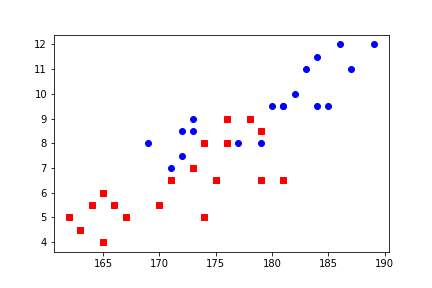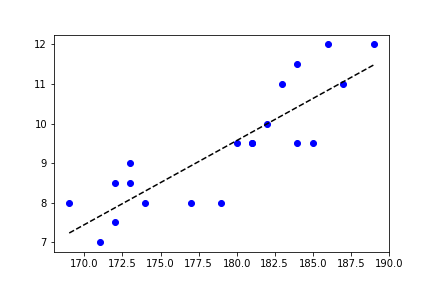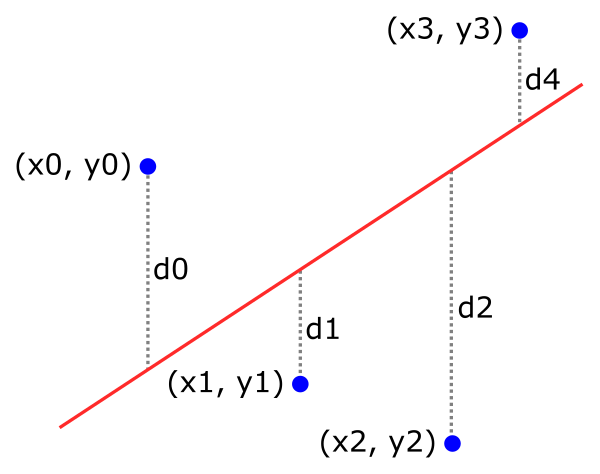# Scatter plots in Matplotlib

Martin McBride, 2021-02-16
Tags numeric python
Categories matplotlib numpyIn this article we will look at scatter plots. A scatter plot is used to compare two variables for a set of data. It can identify relationships, such as correlation, between the variables.

There are two ways to create a scatter plot, The first is to use the plot function, but with the line style set to None, so only the markers appear. If you are creating a simple scatter plot, this method can be slightly faster.

The second way is the scatter function. This allows you to add extra information to your plot by allowing the marker size and/or colour to vary across the graph.

## Scatter plot using the plot function

As a simple example of a scatter plot, for a group of people we might record their height and their shoe size. A scatter plot would then consist of one dot for each person, indicating their height (x) and shoe size (y).

Here is the code to create a scatter plot:

import matplotlib.pyplot as plt

height = [172, 171, 174, 169, 172, 173, 173, 177, 182, 180,
181, 179, 183, 181, 186, 184, 185, 189, 184, 187]
shoe = [8.5, 7.0, 8.0, 8.0, 7.5, 9.0, 8.5, 8.0, 10.0, 9.5,
9.5, 8.0, 11.0, 9.5, 12.0, 11.5, 9.5, 12.0, 9.5, 11.0]

plt.plot(height, shoe, 'bo')
plt.show()


We are using the plot function to create the scatter plot. The key thing here is that the fmt string declares a style 'bo' that indicates colour blue and a round marker, but it doesn't specify a line style. A marker style with no line style turns off line plotting, showing just the markers.

Each (x, y) pair of values corresponds to the height and shoe size of one person in the study. So in the example data, the first person has height 182 cm and shoe size 8.5, the next person has height 171 cm and shoe size 7, and so on. The data uses UK shoe sizes, other countries use a totally different system with very different numbers.

Here is the graph it creates:## Multiple scatter plots

We can plot multiple data sets on the same chart. For example, suppose we had separate sets of height/shoe size data for men and women. We can plot them both like this:

import matplotlib.pyplot as plt

mheight = [172, 171, 174, 169, 172, 173, 173, 177, 182, 180,
181, 179, 183, 181, 186, 184, 185, 189, 184, 187]
mshoe = [8.5, 7.0, 8.0, 8.0, 7.5, 9.0, 8.5, 8.0, 10.0, 9.5,
9.5, 8.0, 11.0, 9.5, 12.0, 11.5, 9.5, 12.0, 9.5, 11.0]

fheight = [165, 164, 167, 162, 165, 163, 166, 170, 175, 173,
174, 171, 175, 174, 179, 176, 178, 181, 176, 179]
fshoe = [4.0, 5.5, 5.0, 5.0, 6.0, 4.5, 5.5, 5.5, 6.5, 7.0,
5.0, 6.5, 6.5, 8.0, 8.5, 9.0, 9.0, 6.5, 8.0, 6.5]

plt.plot(mheight, mshoe, 'bo')
plt.plot(fheight, fshoe, 'rs')
plt.show()


All we need to do is call the plot function twice, once with each set of x, y data. Here is the result:The first set of data is blue circles, the second set is red squares.

## Adding a straight line fit

Returning to the original single data, we will now see how to add a straight line fit to the data

import matplotlib.pyplot as plt

height = [172, 171, 174, 169, 172, 173, 173, 177, 182, 180,
181, 179, 183, 181, 186, 184, 185, 189, 184, 187]
shoe = [8.5, 7.0, 8.0, 8.0, 7.5, 9.0, 8.5, 8.0, 10.0, 9.5,
9.5, 8.0, 11.0, 9.5, 12.0, 11.5, 9.5, 12.0, 9.5, 11.0]

plt.plot(height, shoe, 'bo')
plt.plot([169, 189], [7.23, 11.48], 'k--')
plt.show()


Here is the result. The scatter plot is drawn as before, but we also draw a black, dashed line that represents the best fit of a straight line to the data:### Finding the best fit

This section is optional, it isn't needed to understand Matplotlib, but if you are interested in how the line above was derived, read on.

A common way to find a straight line that fits some scatter data is the least squares method.

For a given set of points (xn, yn) and a line L, for each point you calculate the distance, dn, between the point and the line, like this:We can then calculate the sum of the squares of the distances:

s = d0*d0 + d1*d1 + d2*d2 ...


This sum is a measure of the total error of the line fit. The best line is the one that has the smallest s value.

There is a formula for finding the best fit of a line to a set of (x, y) data points, and fortunately NumPy has an implementation of that formula:

import numpy as np

m, c = np.polyfit(height, shoe, 1)
print(m, c)                # 0.21234550158091434 -28.65607933314174


polyfit takes an array of x-values, and array of y-values, and a polynomial degree. Setting the degree to 1 gives a straight line fit.

The m and c values plug into the standard equation of a straight line:

y = m*x + c


This is a line that crosses the y-axis at c, and has a slope of m. If we plug is a couple of values for x (height 169 and 189), it gives us two points that we can use to draw the line

## Adding an extra dimension with scatter

The scatter function accepts two arrays - the x-values and the y-values - similar to plot. But it does not have a fmt string.

The marker shape is controlled by the marker parameter (which behaves just like the marker parameter of plot). But the other style parameters are controlled by some new variables:

• c - the marker colour. This is similar to the markerfaccolor parameter of plot.
• s - the size of the marker. This is similar to the markersize parameter of plot
• linewidths - the width of the outline around the marker. This is similar to the markeredgewidth parameter of plot
• edgecolors - the colour of the outline of the marker. This is similar to the markeredgecolor parameter of plot

The fun thing about scatter is that you aren't limited to one value for these new variables. You can also supply a sequence of values that are applied to each data point in turn.

### Varying the marker size

We will add an extra dimension to our data - the waist size of each person. This, in our sample, varies between 28 and 44 inches. We will use this value to control the size of each marker.

Here is the code:

import matplotlib.pyplot as plt

height = [172, 171, 174, 169, 172, 173, 173, 177, 182, 180,
181, 179, 183, 181, 186, 184, 185, 189, 184, 187]
shoe = [8.5, 7.0, 8.0, 8.0, 7.5, 9.0, 8.5, 8.0, 10.0, 9.5,
9.5, 8.0, 11.0, 9.5, 12.0, 11.5, 9.5, 12.0, 9.5, 11.0]
waist = [28.0, 30.0, 29.0, 32.0, 30.0, 28.0, 32.0, 29.0, 34.0, 40.0,
32.0, 30.0, 38.0, 40.0, 38.0, 34.0, 42.0, 44.0, 36.0, 36.0]

size = [(x - 26)**2*10 for x in waist]

plt.scatter(height, shoe, s=size, c='b', marker='o', alpha=0.5)
plt.show()


And here is the plotHere, for each data point, the x-axis represents that person's height, the y-axis their shoe size, and the size of each circle indicates their waist measurement. The graph shows an approximate correlation between all 3 values.

We can't use the waist measurement to directly control the marker size, we must first scale it. Here is the scaling formula:

(x - 26)**2*10


We first subtract 26 from the waist value x, to make the value range start at almost zero (the minimum sample is 28). we then square the value, and finally apply a scale factor of 10 to make the markers a reasonable size for the graph.

The reason we need to square this value is because the s parameter is scatter doesn't work in the same as the markersize parameter in plot:

• markersize (in the plot function) controls the width of the marker.
• s (in the scatter function) controls the area of the marker.

This means that if we plot a marker in scatter with an s value of 1, and another marker with an s value of 9, the second marker has 9 times the area of the first. It is only 3 times the width. If you want the second marker to be 9 times the width, you must give it an s value of 81 (ie 9 squared).

There is no right or wrong way to do it. You can choose whichever way you feel best represents your data.

If you found this article useful, you might be interested in the course Introduction to Matplotlib by the same author.

#### Popular tags

2d arrays abstract data type alignment and angle animation arange arc array arrays behavioural pattern bezier curve built-in function callable object chain circle classes clipping close closure cmyk colour combinations comparison operator comprehension context context manager conversion count creational pattern data science data types design pattern device space dictionary drawing duck typing efficiency ellipse else encryption enumerate fill filter font font style for loop function function composition function plot functools game development generativepy tutorial generator geometry gif global variable gradient greyscale higher order function hsl html image image processing imagesurface immutable object in operator index inner function input installing iter iterable iterator itertools join l system lambda function len line linear gradient linspace list list comprehension logical operator lru_cache magic method mandelbrot mandelbrot set map matplotlib monad mutability named parameter numeric python numpy object open operator optimisation optional parameter or pandas partial application path pattern permutations polygon positional parameter print pure function python standard library radial gradient range recipes rectangle recursion reduce repeat rgb rotation roundrect scaling scipy sector segment sequence setup shape singleton slice slicing sound spirograph sprite square str stream string stroke structural pattern subpath symmetric encryption template text text metrics tinkerbell fractal transform translation transparency triangle truthy value tuple turtle unpacking user space vectorisation webserver website while loop zip